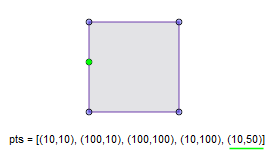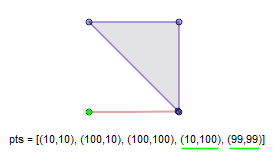# CleanPolygon

Del.» function CleanPolygon(const Poly: TPath; Distance: double = 1.415): TPath;

C++ » void CleanPolygon(const Path &in_poly, Path &out_poly, double distance = 1.415);

C++ » void CleanPolygon(Path &poly, double distance = 1.415);

C#  » public static Path CleanPolygon(Path poly, double distance = 1.415);

Removes vertices:

• that join co-linear edges, or join edges that are almost co-linear (such that if the vertex was moved no more than the specified distance the edges would be co-linear)
• that are within the specified distance of an adjacent vertex
• that are within the specified distance of a semi-adjacent vertex together with their out-lying vertices

Vertices are semi-adjacent when they are separated by a single (out-lying) vertex.

The distance parameter's default value is approximately √2 so that a vertex will be removed when adjacent or semi-adjacent vertices having their corresponding X and Y coordinates differing by no more than 1 unit. (If the egdes are semi-adjacent the out-lying vertex will be removed too.)

C++ only: This function is overloaded. In the first definition, the in_poly and out_poly parameters can reference the same Path object though in that case the calling code might be clearer if the second definition (accepting a single Paths parameter) is used.